### IMO Shortlist 1996 problem G5

Kvaliteta:
Avg: 0,0
Težina:
Avg: 8,0
Let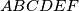$ABCDEF$ be a convex hexagon such that$AB$ is parallel to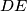$DE$,$BC$ is parallel to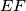$EF$, and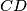$CD$ is parallel to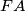$FA$. Let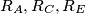$R_{A},R_{C},R_{E}$ denote the circumradii of triangles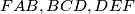$FAB,BCD,DEF$, respectively, and let$P$ denote the perimeter of the hexagon. Prove that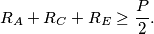Izvor: Međunarodna matematička olimpijada, shortlist 1996